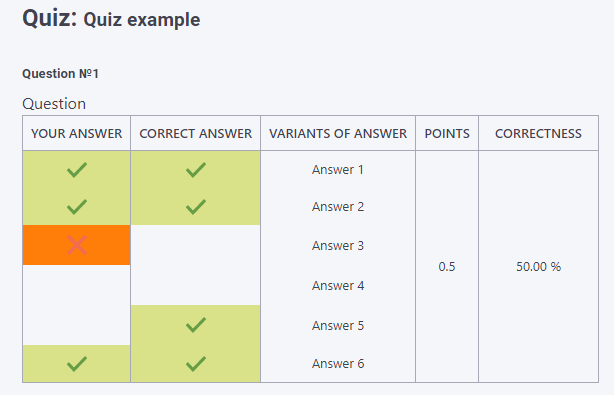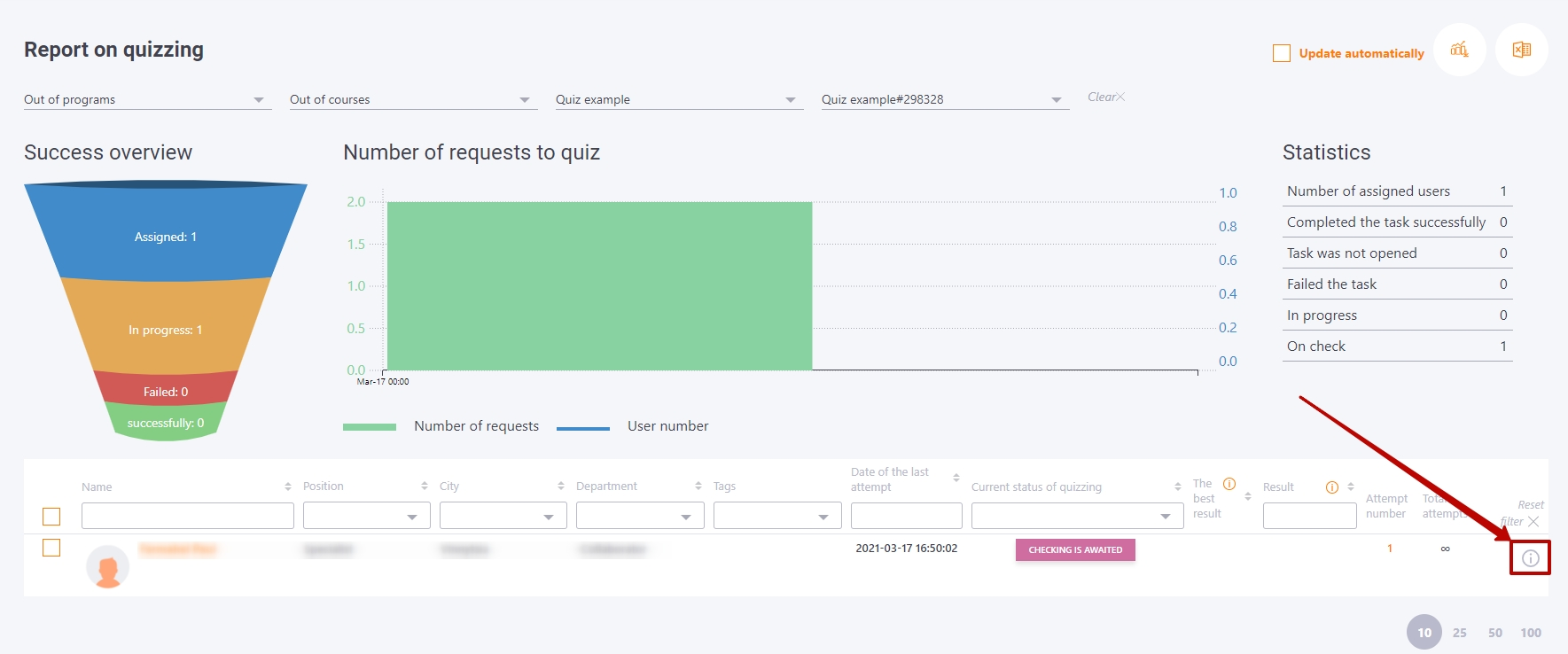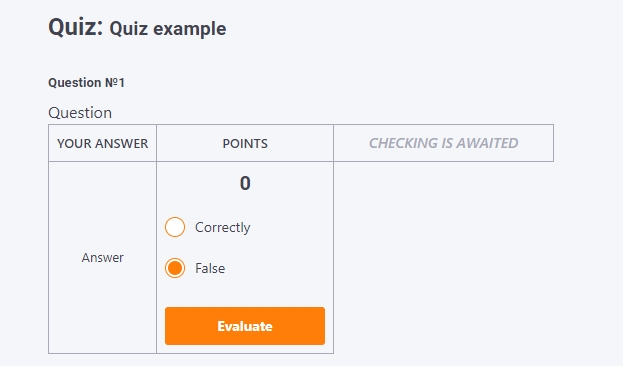# LMS Collaborator knowledge base

Home/Content/Quizzes/Calculation of results for quizzes

## Calculation of results for quizzes

Each question of the quiz has its own weight W in points.

When a quiz is given to a user, a sample of N questions is generated. An absolutely correct answer to all questions will give the user the maximum sum of all question weights, i.e. Wmax = W1 max + W2 max + ... + Wn max.

The result of the quiz will be a % of the maximum possible mark:

Quiz = (W1 + W2 + ... + Wn) * 100% / Wmax

Multiple

In the answer to such a question, the user is offered to choose more than one variant.

Among the proposed variants there are correct (denoted by 1) and wrong (denoted by 0). For example, if a question contains Nfull=10 answer variants, among which Np=5 are correct and Nn=5 are wrong, it can be represented as a vector

1
1
1
1
1
0
0
0
0
0

When answering a question, the user marks the variants that they consider correct.

We compare two vectors of answers: the user and the template given in the question. 1 - ticked, 0 - not ticked
We get something like this (10 answer variants):

User Benchmark Correct choice Wrong choice
1 1 1 0
1 1 1 0
1 1 1 0
0 1 0 1
0 1 0 1
0 0 0 0
0 0 0 0
0 0 0 0
1 0 0 1
1 0 0 1

The result for answering such a question is calculated as follows:

``    Rez = (A - B) * W * 100% / Np``

A - number of correct choices
B - number of wrong choices
W - question weight
Np - the number of correct choices in the question (example = 5)
N - the number of all choices in the question (example = 10)

The multiple question type has the parameter Nk "Number of answers that can be selected.
It can be set from Np and N. By default it is equal to the number of correct choices Np. I.e.
Nk >= Np
Result calculation algorithm:

``````if (A - B) <= 0
Rez = 0
otherwise
Rez = (A - B) * W * 100% / Np;``````

#### Example

For example, if there is such a question with variants of answers for which W = 1 and Np = 2:

```Which of these is eatable?
( ) Stone
( ) Nail
( ) Wood
(+) Cucumber
(+) Sugar
```

Answer variants and the final result on the answer to the question:

```   ( ) Stone   ( ) Stone    (+) Stone    ( ) Stone
( ) Nail  ( ) Nail    (+) Nail    ( ) Nail
(+) Wood   (+) Wood      (+) Wood       ( ) Wood
(+) Cucumber  ( ) Cucumber    (+) Cucumber    (+) Cucumber
(+) Sugar    (+) Sugar     (+) Sugar      (+) Sugar
А = 2        А = 1         А = 2         А = 2
B = 1        B = 1         B = 3         B = 0
[A-B]=1      [A-B]=0       [A-B]=-1      [A-B]=2
Rez = 50%    Rez = 0%      Rez = 0%      Rez = 100%
```

#### Example 2

The quiz has a multiple choice question. There are 6 variants, of which 4 are correct. There is a limit of 4 possible answers.
The user will pick 4 answers - 3 correct and 1 incorrect. The result for this question will be 0.5.The user could have chosen 3 correct variants and stopped there, then his score would have been 0.75.
But he chose one more option and got it wrong.

1. did not choose (did not know) one correct variant - and did not get 1/4 points to the maximum score,
2. chose (did not know) the wrong variant - received a penalty for an error of 1/4 point.

### Classification question

The answer to this type of question asks you to assign the answer variant terms to the given classes. As with a Multiple choice question the user can select multiple correct and multiple wrong answers.

When the user saves the choice, the number of correct answers is counted as A.

An absolutely (100%) correct answer is one in which all terms are correctly assigned to classes, then the number of correct answers is N (equal to the number of all answers).

The algorithm for calculating the result of an answer to a question with the weight W:

``   Rez = A * W * 100% / N;``

### Ordering question

In this type of question, you must put the answer variants in the correct order.

The result is calculated in the same way as for the classification questions:

```
Rez = A * W * 100% / N;
where
А - number of correct answers,
W - question weight,
N - total number of answers.
```

The quiz will change to status Completed or Failed only if all of the answers to the free answers are scored. It's important for quizzes with more than one free answer.

### Evaluation of free-answer quizzes if the user has used SEVERAL ATTEMPTS

The quiz result depends on the task setting Evaluation and awards (Find more in Evaluation and awards)

• the result of the last attempt

It is important to check the user's answers for the last attempt to pass the quiz in order to get the results for the Administrator

• the best result among all attempts

It is important to check the answers to all attempts in order to get the results of the quiz for the Administrator. Only after checking all the answers can the system determine which attempt was better.

Detailed attempt reports can be viewed in the Quizzes and Complex Quizzes reports